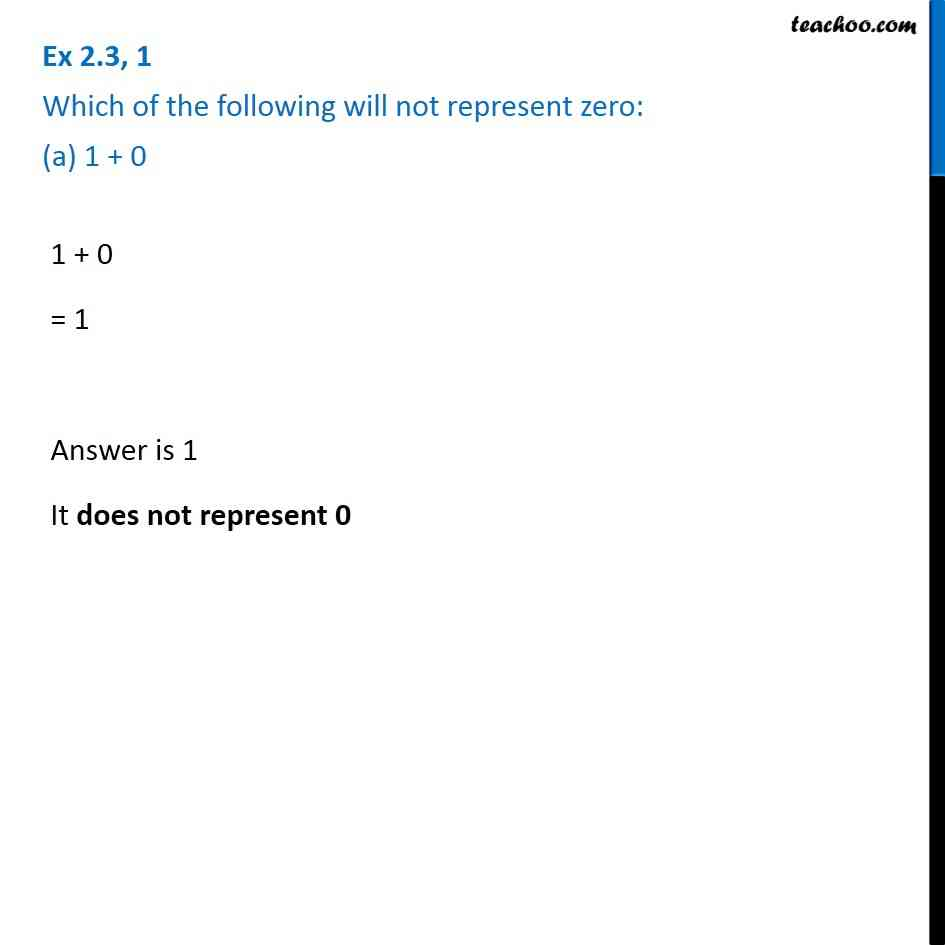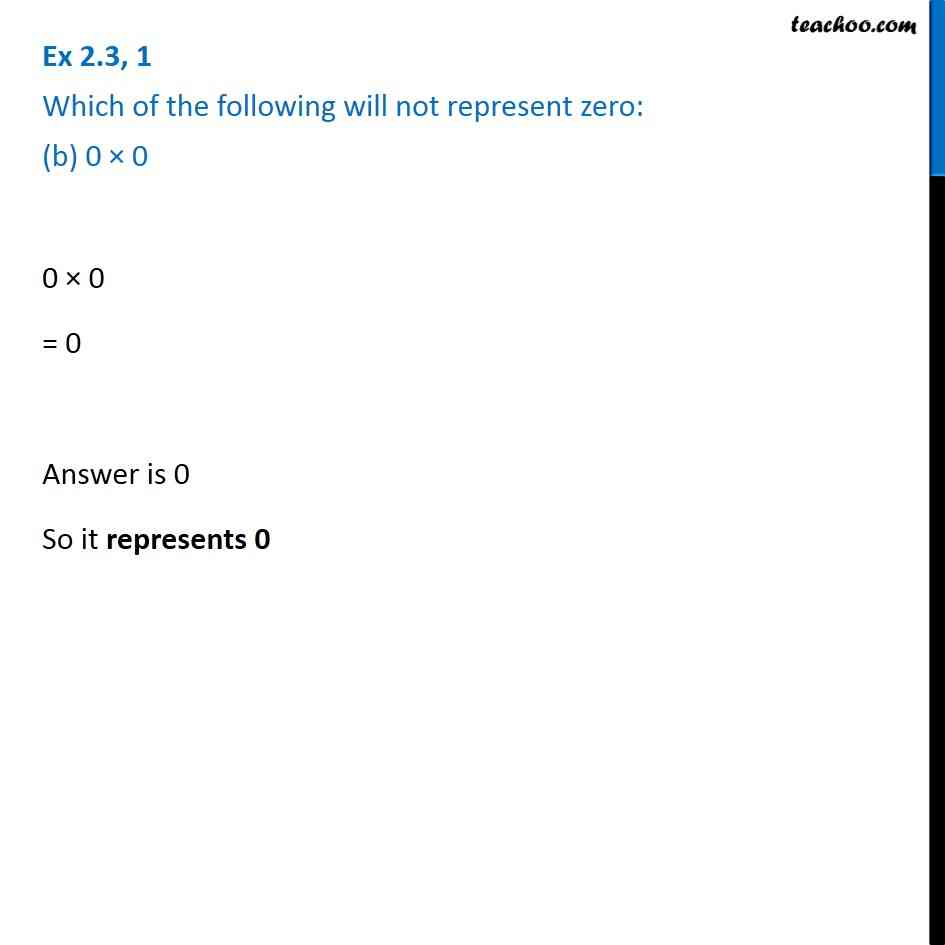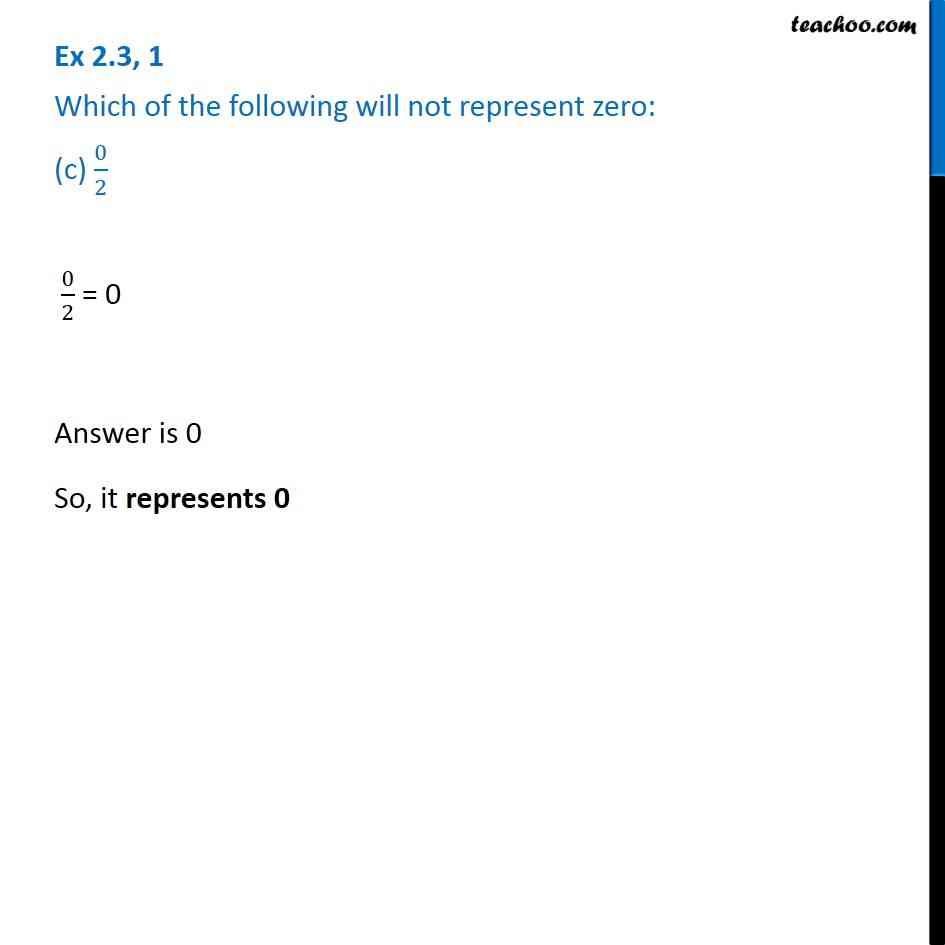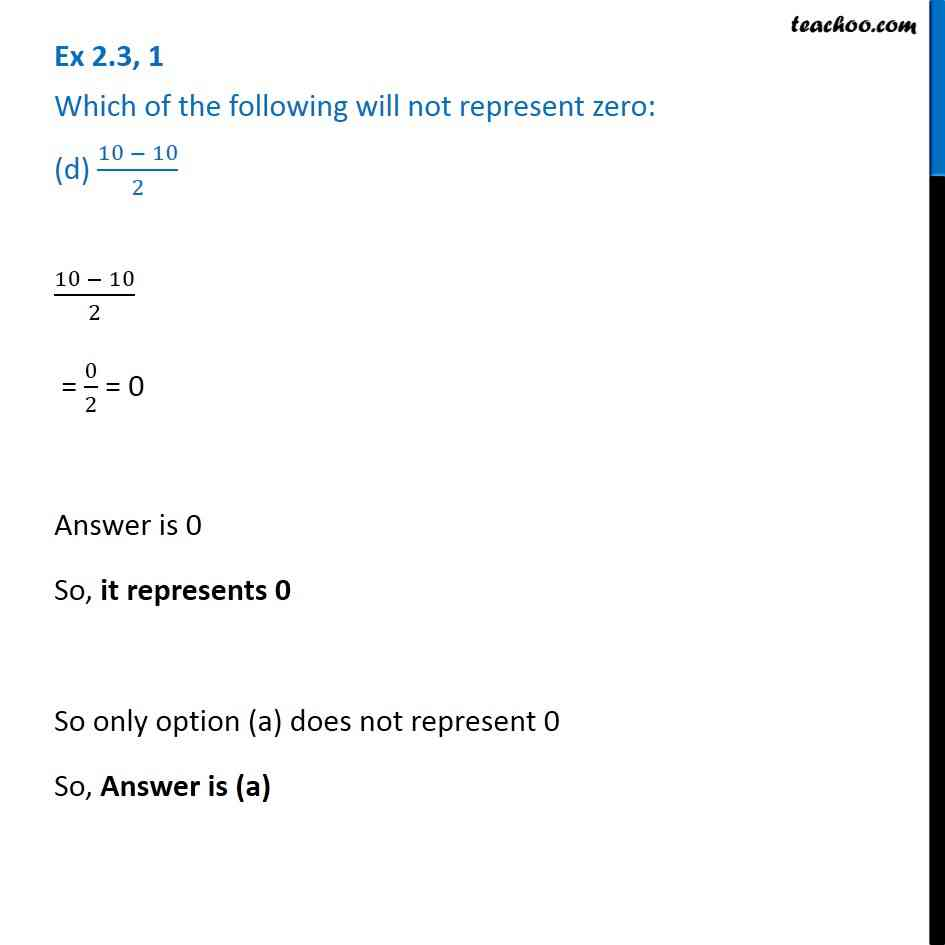Properties of Whole Numbers

Chapter 2 Class 6 Whole Numbers
Serial order wiseLearn in your speed, with individual attention - Teachoo Maths 1-on-1 Class

### Transcript

Question 1 Which of the following will not represent zero: (a) 1 + 0 1 + 0 = 1 Answer is 1 It does not represent 0 Question 1 Which of the following will not represent zero: (b) 0 × 0 0 × 0 = 0 Answer is 0 So it represents 0 Question 1 Which of the following will not represent zero: (c) 0/2 0/2 = 0 Answer is 0 So, it represents 0 Question 1 Which of the following will not represent zero: (d) (10 - 10)/2 (10 - 10)/2 = 0/2 = 0 Answer is 0 So, it represents 0 So only option (a) does not represent 0 So, Answer is (a)